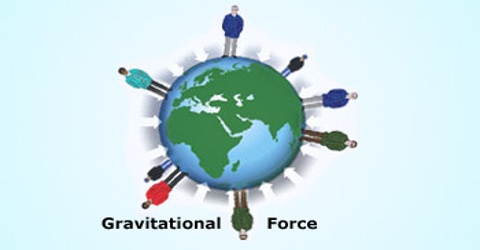# Idea of Force: Gravitational and Gravity Force

Idea of Force: Gravitational and Gravity Force

Scientist Sir Isaac Newton first discovered the force of attraction between two bodies. In reality each body has a fixed size. When the distance between two extended bodies is much larger than their sizes then and then we can consider them as particles ignoring their sizes. In this case mass of each body is considered to be concentrated the centre and by applying Newton’s gravitational law attractive force between them is determined. This force of attraction between the two bodies in the universe as called gravitational force. Sometimes this force is celled gravity force or force of gravity of the two bodies. If one is the earth then that attraction between the two bodies is the gravity force. The force by which the earth attracts a body to centre is called the weight of that body. That means weight of the force of gravity on that body.If the mass of the body is m, its weight is g, then,

W = mg.

Weight = mass x acceleration due to gravity.

Weight of a body also acts vertically downward i.e., acts towards the centre of the earth. Weight is a vector quantity. Weight of a body is variable. If the value of acceleration due to gravity changes then weight also changes proportionately.

Example: Force between the earth and a mango is gravity whereas force between two mangoes is gravitational.